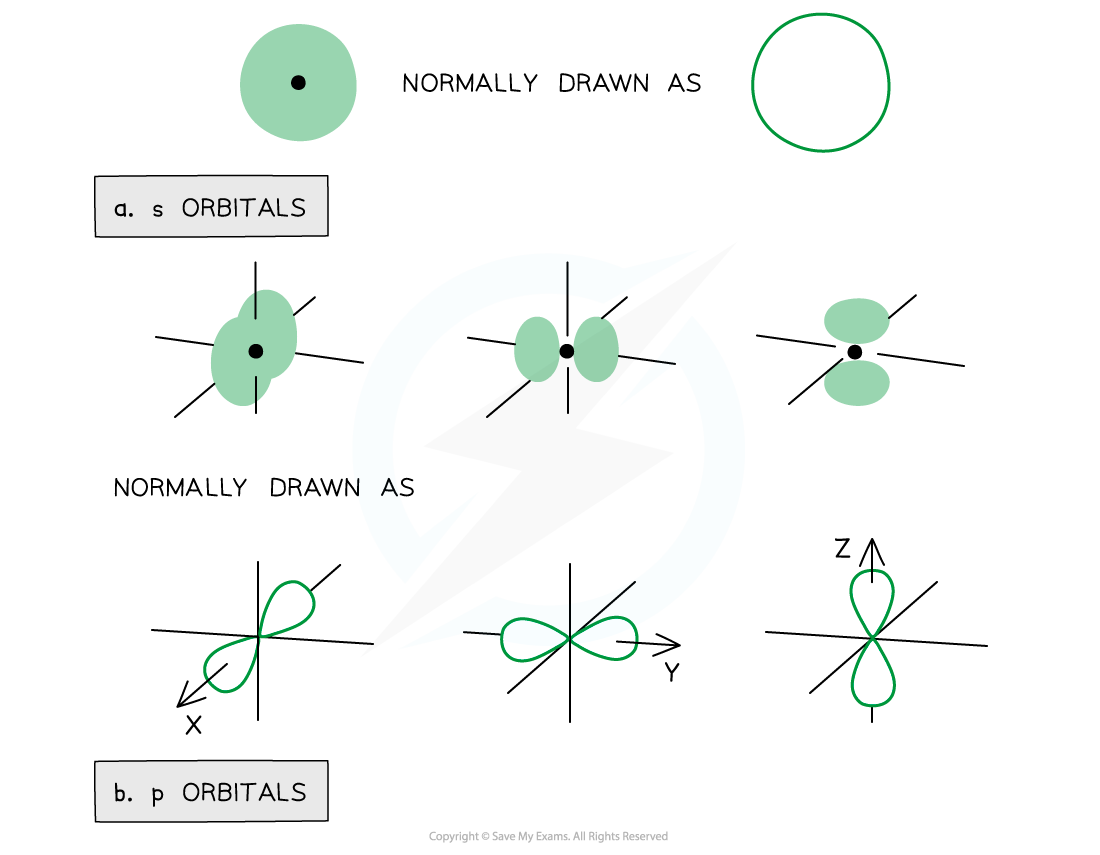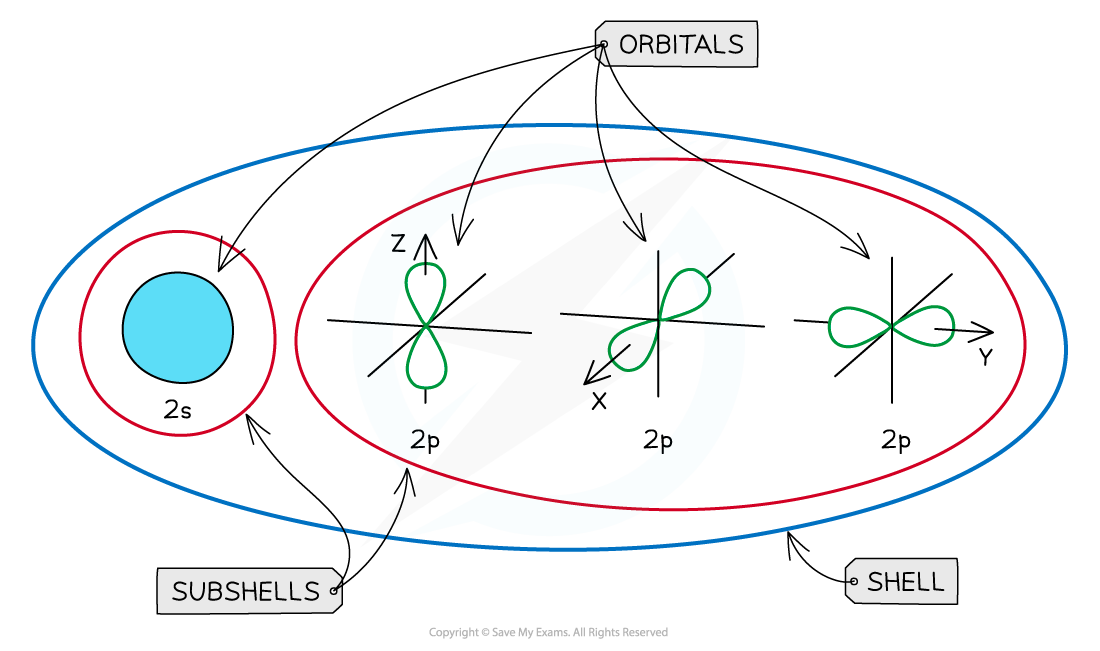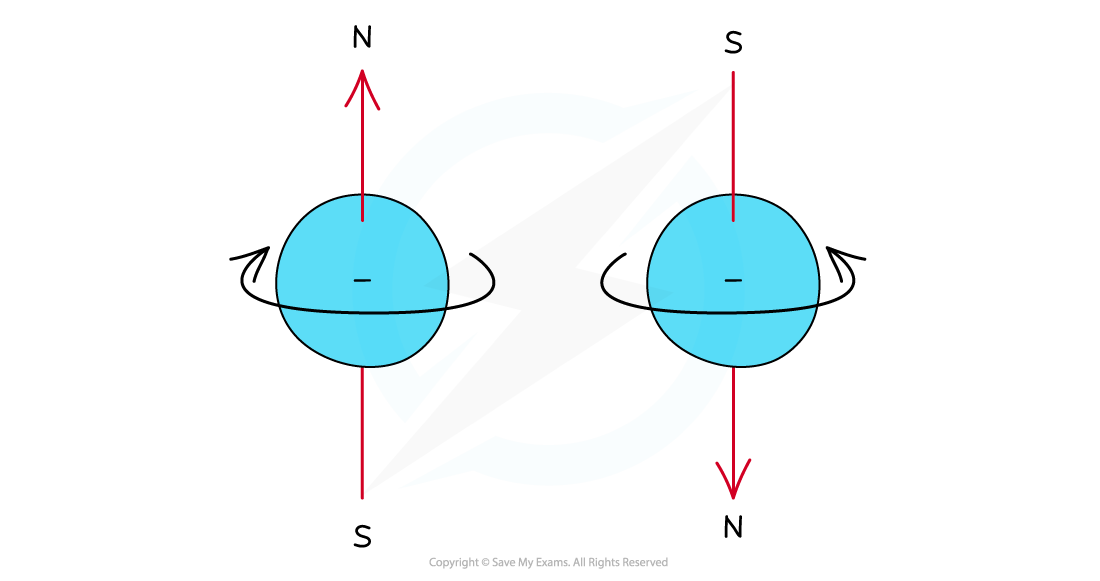# Edexcel A Level Chemistry:复习笔记1.2.3 Orbitals

### Orbital Shapes

#### Orbitals

• Subshells contain one or more atomic orbitals
• Orbitals exist at specific energy levels and electrons can only be found at these specific levels, not in between them
• Each atomic orbital can be occupied by a maximum of two electrons

• This means that the number of orbitals in each subshell is as follows:
• s : one orbital (1 x 2 = total of 2 electrons)
• p : three orbitals ( 3 x 2 = total of 6 electrons)
• d : five orbitals (5 x 2 = total of 10 electrons)
• f : seven orbitals (7 x 2 = total of 14 electrons)

• The orbitals have specific 3-D shapes

s orbital shape

• The s orbitals are spherical in shape
• The size of the s orbitals increases with increasing shell number
• E.g. the s orbital of the third quantum shell (n = 3) is bigger than the s orbital of the first quantum shell (n = 1)

#### p orbital shape

• The p orbitals have a dumbbell shape
• Every shell has three p orbitals except for the first one (n = 1)
• The p orbitals occupy the x, y and z axes and point at right angles to each other, so are oriented perpendicular to one another
• The lobes of the p orbitals become larger and longer with increasing shell numberRepresentation of orbitals (the dot represents the nucleus of the atom) showing spherical s orbitals (a), p orbitals containing ‘lobes’ along the x, y and z axis

• Note that the shape of the d orbitals is not requiredAn overview of the shells, subshells and orbitals in an atom

### Filling Orbitals

• Electrons can be imagined as small spinning charges which rotate around their own axis in either a clockwise or anticlockwise direction
• The spin of the electron is represented by its direction
• The spin creates a tiny magnetic field with N-S pole pointing up or downElectrons can spin either in a clockwise or anticlockwise direction around their own axis

• Electrons with the same spin repel each other which is also called spin-pair repulsion
• Therefore, electrons will occupy separate orbitals in the same subshell first to minimise this repulsion and have their spin in the same direction
• They will then pair up, with a second electron being added to the first p orbital, with its spin in the opposite direction

• This is known as Hund's Rule
• E.g. if there are three electrons in a p subshell, one electron will go into each px, py and pz orbitalElectron configuration: three electrons in a p subshell

• The principal quantum number indicates the energy level of a particular shell but also indicates the energy of the electrons in that shell
• A 2p electron is in the second shell and therefore has an energy corresponding to n = 2

• Even though there is repulsion between negatively charged electrons, they occupy the same region of space in orbitals
• An orbital can only hold two electrons and they must have opposite spin - the is known as the Pauli Exclusion Principle
• This is because the energy required to jump to a higher empty orbital is greater than the inter-electron repulsion
• For this reason, they pair up and occupy the lower energy levels first